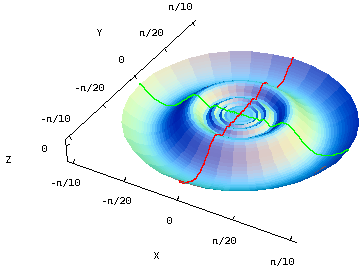Math Insight

Applet: A differentiable function with discontinuous partial derivativesThe function $$f(x,y)=\begin{cases}(x^2+y^2)\sin\left(\frac{1}{\sqrt{x^2+y^2}}\right) & \text{ if (x,y) \ne (0,0)}\\0 & \text{ if (x,y) = (0,0)}\end{cases}$$ is differentiable even at the origin. You can see this because $f(x,y)$ is squeezed between the two elliptic paraboloids $z=x^2+y^2$ and $z=-x^2-y^2$, both of which share the horizontal tangent plane $z=0$ at the origin.

The cross sections $x=0$ (in red) and $y=0$ (in green) also highlight the how the graph flattens out and stays differentiable at the origin despite the rapid oscillations of the sinusoid. You can press “F” when your mouse is over the graph to remove the “faces” composing the surface and see the cross sections in isolation.

The partial derivatives at the origin are zero, as they must be given the horizontal tangent plane. (You have to use the limit definition to calculate them.) The partial derivatives oscillate wildly as one approaches the origin, getting arbitrarily close to both 1 and $-1$ within any neighborhood of the origin. Therefore, the partial derivatives are discontinuous at the origin.

This function provides a counterexample showing that partial derivatives do not need to be continuous for a function to be differentiable, demonstrating that the converse of the differentiability theorem is not true.

This applet is found in the pages

List of all applets